## Quiz 15 : ForecastingLooking for Decision Science Homework Help?# Quiz 15 : Forecasting

BEI airport has a problem in staffing and security checking. In the peak season it is having the problem of long waiting lines and waiting times. BEI decided to forecast the airport passenger arrival for the next year by using the past 3 year's data. Airlines run for every two hours' time segment from 4:00 A.M to 10:00 P.M and the demand for airline travel has been increasing from the last 3 years. The forecast for the next year July can be calculated using time series analysis method of forecasting, using Moving average method of demand forecasting. The formula for computing the simple moving average forecasting is as follows:The following table is the forecast of airline passengers for 10 days in the month of July calculated through moving average method of demand forecasting.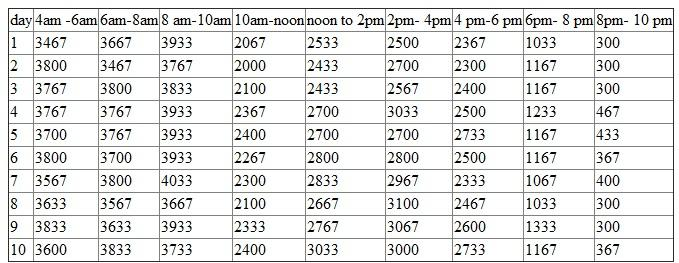From the above table it is observed that there is a heavy flow of passengers from 4:00 A.M to 10:00 A.M. hence, more checkpoints are needed at this time segments.

Forecasting is an integral part of every organization as it helps to estimate many future aspects of a business or other operation. As per the stated case, SU is facing cash crunch and deficit in resources such as classroom, faculty, and dormitory rooms. In such a scenario, the company can use the forecasting methods effectively to ease up the economic situation. Some of the forecasting techniques are listed below: Qualitative method : This is a method that relies on experts or manager's opinion in making future predictions. Besides that, it is useful for medium to long range forecasting tasks. Some of the qualitative methods are listed below: • Delphi Technique : It is a technique where forecast is developed through group consensus. Experts are given questionaries' and are asked about their suggestions and opinions on the underlying issue. • Market surveys : It involves the use of consumer panels, questionnaire, tests of new products and services. • Scenario writing : It is a process wherein possible future events are analyzed by considering alternative possible outcomes. Another important method is quantitative technique that helps in making efficient forecasting. It is a method wherein the future demand is predicted from the past data and extrapolated to make forecasts of future levels. Some of the widely used quantitative techniques are trend analysis, moving average method, exponential smoothening, and weighted average method. Hence, these methods can help SU to meet the shortage of resources and implementing the management practices appropriately.

The Moving Average Forecast method uses the data from the past periods to forecast the value of the variable for the future periods. It averages the data from the most recent past periods to forecast the future value of the variable. This method tends to smoothed out the arbitrary increase or decrease of forecast. The moving average forecast is utilized when demand data does not exhibit any trend or seasonality. a. 3 - Month Moving Average Forecast A 3 - month moving average forecast for a period is calculated by averaging the demand over the last three months. Use the following formula to calculate a 3 - month moving average forecast.Where, MA 3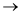3 - month moving average DiDemand in period i iperiod i (month) The following table shows the 3-month moving average forecast for April through January (of the next year):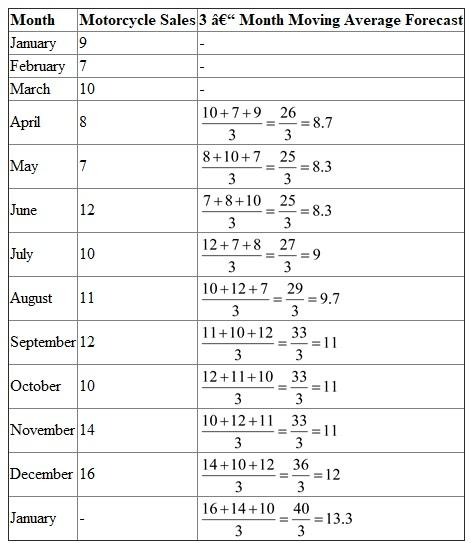b. The 5 - month moving average forecast is similar to the 3 - month moving average forecast. In 5 - month moving average forecast, the demand data from the recent past 5 - months is averaged to compute the forecast. Use the following formula to compute the 5 - month moving average forecast.Where, MA 55-month moving average DiDemand in period i iperiod i (month) The following table shows the 5-month moving average forecast for June through January (of the next year):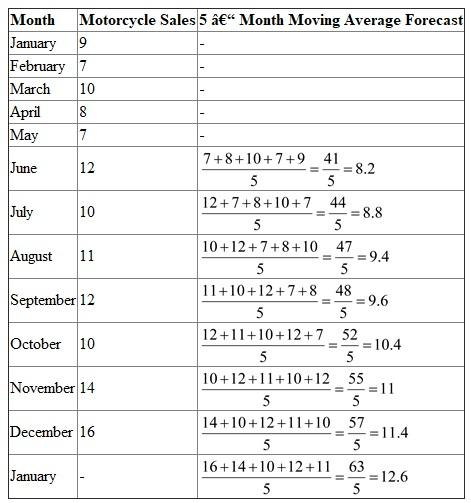c. The Mean Absolute Deviation Error (MAD) is the forecast error calculated by computing the average of the absolute deviation between the forecasted demand and the actual demand. Use the following formula to compute the Mean Absolute Deviation Error.Where, MADMean Absolute Deviation DtActual demand in period t FtForecasted demand in period t tPeriod t (month) nNumber of periods The following table shows the Mean Absolute Deviation Error (MAD) for the 3 - month moving average forecast: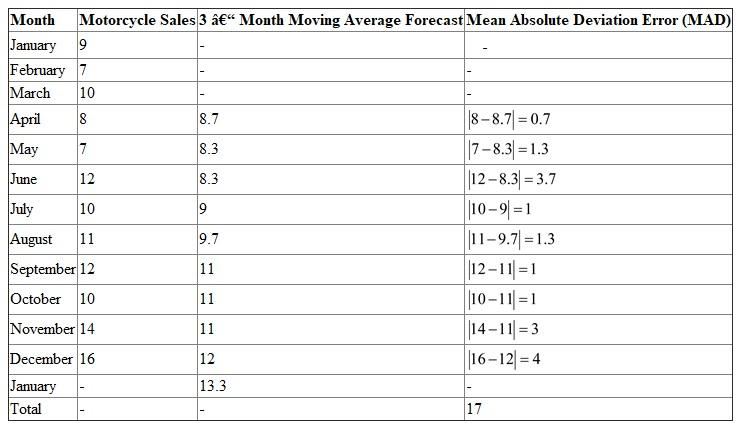Mean Absolute Deviation (MAD) error for 3 - month moving average forecast is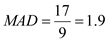Mean Absolute Deviation (MAD) error for 3 - month moving average forecast is 1.9 The following table shows the Mean Absolute Deviation Error (MAD) for the 5 - month moving average forecast: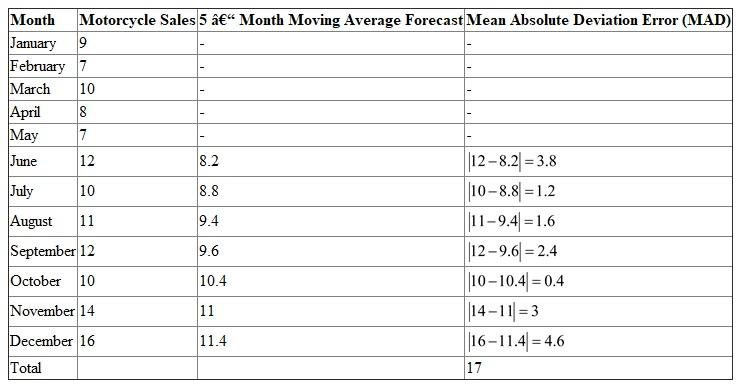Mean Absolute Deviation (MAD) error for 5 - month moving average forecast is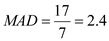Mean Absolute Deviation (MAD) error for 5 - month moving average forecast is 2.4 The forecast with lower value of MAD is more accurate, therefore the forecast for 3 - month moving average is more accurate than 5 - month moving average.

There is no answer for this question